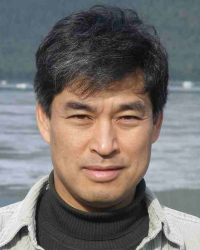Mathematicothermodynamics
Speaker
Prof. Hong Qian
University of Washington, Seattle
Abstract

There is a growing awareness toward a slow shifting in the foundation of the thermodynamic laws, from several macroscopic, empirical postulates concerning heat as a form of random motions, to derivable mathematical theorems based on stochastic dynamics of mesoscopic systems.  It becomes increasingly clear that a stochastic dynamic description of the Nature is a very effective mathematical representation of the Reality.  In this talk, I shall first introduce this mathematicothermodynamics as a set of mathematical results, and then apply them to two significant problems: (1) The Gibbsian macroscopic isothermal chemical thermodynamics via a mathematical limit by merely allowing the molecular numbers to be infinite; (2) A lift of a finite Markov process with irreversible cycles to an infinite system that establishes, in the long-time limit, the entropy production in the former is equal to the potential energy decrease in the latter.  This result unifies the Clausius' and Kelvin' s statements of the Second Law.